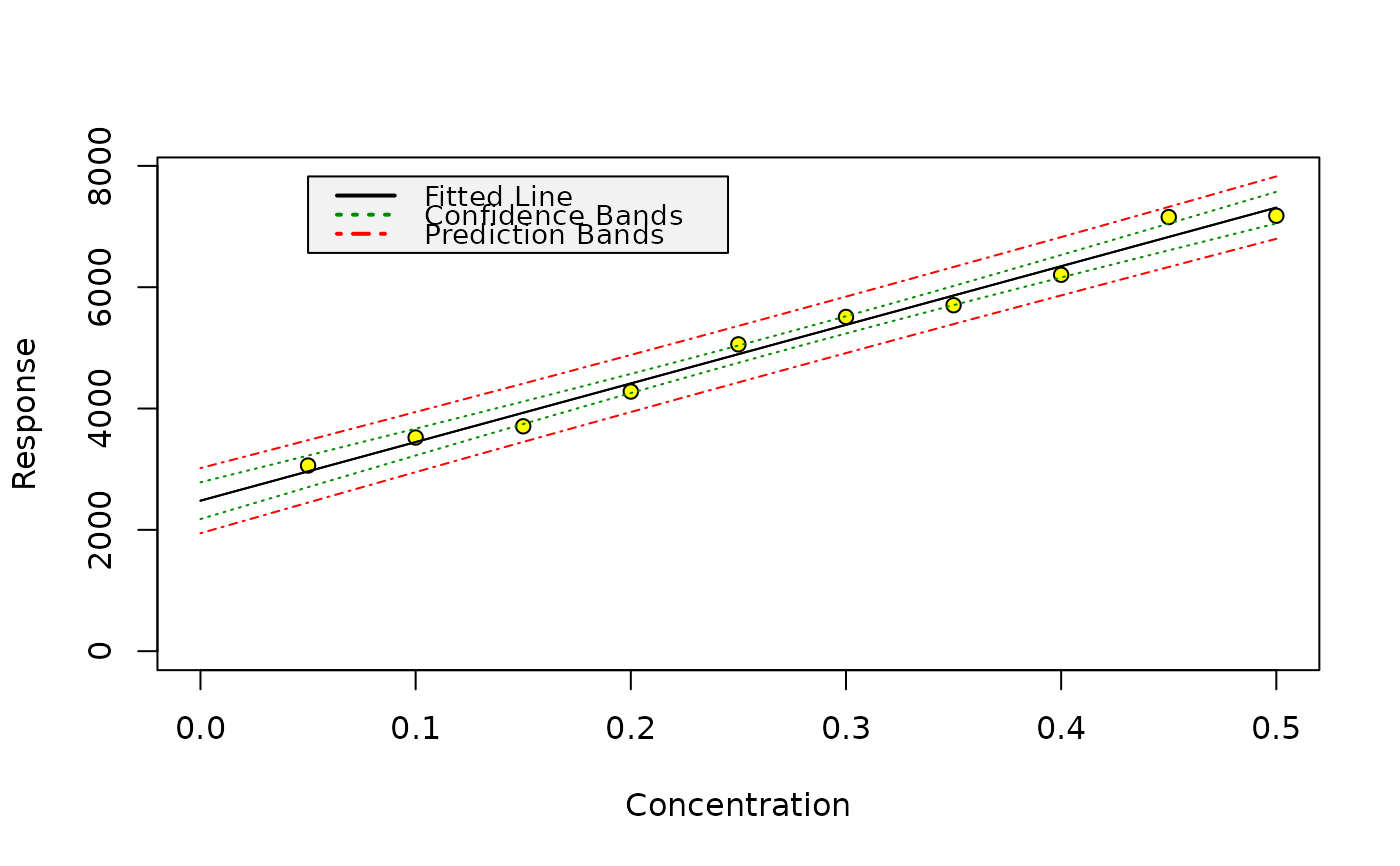Sample dataset to test the package.

## Format

A dataframe containing 10 rows of x and y values.

## Examples


m <- lm(y ~ x, data = din32645)
calplot(m)## Prediction of x with confidence interval
prediction <- inverse.predict(m, 3500, alpha = 0.01)

# This should give 0.07434 according to test data from Dintest, which
# was collected from Procontrol 3.1 (isomehr GmbH) in this case
round(prediction$Confidence, 5) #>  0.07434 ## Critical value: crit <- lod(m, alpha = 0.01, beta = 0.5) # According to DIN 32645, we should get 0.07 for the critical value # (decision limit, "Nachweisgrenze") round(crit$x, 2)
#>  0.07
# and according to Dintest test data, we should get 0.0698 from
round(crit$x, 4) #>  0.0698 ## Limit of detection (smallest detectable value given alpha and beta) # In German, the smallest detectable value is the "Erfassungsgrenze", and we # should get 0.14 according to DIN, which we achieve by using the method # described in it: lod.din <- lod(m, alpha = 0.01, beta = 0.01, method = "din") round(lod.din$x, 2)
#>  0.14

## Limit of quantification
# This accords to the test data coming with the test data from Dintest again,
# except for the last digits of the value cited for Procontrol 3.1 (0.2121)
loq <- loq(m, alpha = 0.01)
round(loq$x, 4) #>  0.212 # A similar value is obtained using the approximation # LQ = 3.04 * LC (Currie 1999, p. 120) 3.04 * lod(m, alpha = 0.01, beta = 0.5)$x
#>  0.2122306